# Chapter 16: Color Spaces, Patterns and Shadings

## 16.1 PdfColorSpace Object

16.1.1 Color Space Overview

The PDF format offers various methods for specifying the colors of graphics objects to be painted on the current page. Colors can be described in any of a variety of color systems, or color spaces. Some color spaces are related to device color representation (grayscale, RGB, CMYK), others to human visual perception (CIE-based.)

In PDF, a color space is an important component of the current graphics state along with other drawing parameters such as stroking and filling colors, line width, and others. Versions of AspPDF prior to 2.5 only supported RGB and CMYK color spaces via the PdfCanvas method SetColor/SetFillColor and SetColorCMYK/SetFillColorCMYK. These methods implicitly set the current color space to RGB and CMYK, respectively, and then specify the current color within this color space.

As of Version 2.5, AspPDF provides separate methods for specifying the current color space, and current color: SetColorSpace/SetFillColorSpace and SetColorEx/SetFillColorEx. This version also offers support for many more color spaces in addition to RGB and CMYK: Indexed, Lab, ICC-based, Separation, and others.

NOTE: A detailed discussion of PDF color spaces is outside the scope of this user manual. Please refer to the Adobe PDF Reference for detailed information on this vast subject.

16.1.2 PdfColorSpace Object Usage

To create an instance of the PdfColorSpace object, the PdfDocument method CreateColorSpace should be called. This method expects two arguments: the color space name, and an optional list of parameters. The valid color space names are: "DeviceRGB", "DeviceCMYK", "DeviceGray", "CalRGB", "CalGray", "Lab", "ICCBased", "Indexed", "Separation" and "DeviceN".

When the newly created PdfColorSpace object represents a basic color space such as "DeviceRGB", no additional steps are required and the object is ready to be used. However, if the color space is an advanced one, some additional properties and/or methods need to be called on the PdfColorSpace object for it to be valid and complete, as shown below. For debugging purposes, the PdfColorSpace object exposes the property IsValid which returns True if this object is valid and complete, and False otherwise. The exact verbal reason for the object not being valid should be retrieved via the property ValidationError.

Once a valid instance of the PdfColorSpace object is created, it can be used on the canvas of a page or graphics via the PdfCanvas methods SetColorSpace and SetFillColorSpace to set the new active color space for stroking and fill operations, respectively.

Once a color space is made active, the current color within this color space should be specified via the methods SetColorEx and SetFillColorEx for stroking and fill operations, respectively. These methods expect a single argument, a PdfParam object or parameter string through which all color components c1, c2, ..., cN are specified.

For example, the following sequence creates an RGB color space, activates it for stroking operations and sets the current stroking color to (0.5, 0.7, 1):

Set csRGB = Doc.CreateColorSpace("DeviceRGB")
Page.Canvas.SetColorSpace csRGB
Page.Canvas.SetColorEx "c1=0.5; c2=0.7; c3=1"

Note that the sequence above could be replaced by a single line of code:

Page.Canvas.SetColor 0.5, 0.7, 1

The following sequence creates an CMYK color space, activates it for fill operations and sets the current fill color to (0.3, 0.5, 0.8, 0.2):

Set csCMYK = Doc.CreateColorSpace("DeviceCMYK")
Page.Canvas.SetFillColorSpace csCMYK
Page.Canvas.SetFillColorEx "c1=0.3; c2=0.5; c3=0.8; c4=0.2"

The sequence above has the same effect as:

Page.Canvas.SetFillColorCMYK 0.3, 0.5, 0.8, 0.2

Note that it is the responsibility of the developer to ensure the correct number of color components are passed to the SetColorEx and SetFillColorEx methods in accordance with the currently active color space (e.g. 3 for RGB, 4 for CMYK, 1 for grayscale, etc.)

In addition to the use with the SetColorSpace and SetFillColorSpace methods of the PdfCanvas object, a color space object can also be assigned to an image via the method PdfImage.SetColorSpace.

16.1.3 Supported Color Spaces

This sub-section describes all color spaces currently supported by AspPDF, and the parameters and steps required to create a PdfColorSpace object representing each one.

DeviceRGB, DeviceCMYK, DeviceGray

These device color spaces enable a page description to specify color values that are directly related to their representation on an output device.

To create an RGB, CMYK or Grayscale device color space, the CreateColorSpace method must be called with the string "DeviceRGB", "DeviceCMYK", and "DeviceGray" as the first argument, respectively. The 2nd argument should be omitted as these color spaces require no additional parameters. No additional steps are necessary.

The number of color components for these color spaces are 3, 4 and 1, respectively.

Examples:

Set csRGB = Doc.CreateColorSpace("DeviceRGB")

Set csCMYK = Doc.CreateColorSpace("DeviceCMYK")

Set csGray = Doc.CreateColorSpace("DeviceGray")

CalGray

Calibrated color in PDF is defined in terms of an international standard used in the graphic arts, television and printing industries. CIE-based color spaces enable a page description to specify color values in a way that is related to human visual perception. The CalGray color space (as well as CalRGB, Lab and ICCBased described below) is a CIE-based color space. It has a single color component.

To create a CalGray color space, the CreateColorSpace method must be called with the string "CalGray" as the first argument. The 2nd argument must be set to a PdfParam object or parameter string specifying CalGray-specific parameters. These are:

• Xw, Yw, Zw (required) - the diffuse white point. Xw and Zx must be positive and Yw must be equal to 1.
• Xb, Yb, Zb (optional) - the diffuse black point. All three must be non-negative. (0, 0, 0) by default.
• Gamma (optional) - gamma for the gray component. Must be positive, generally greater or equal to 1. 1 by default.

Example:

Set Param = PDF.CreateParam
Param("Xw") = 0.95
Param("Yw") = 1
Param("Zw") = 1.089
Param("Gamma") = 2.222
Set csCalGray = doc.CreateColorSpace("CalGray", Param)

CalRGB

CalRGB is another CIE-based color space which has 3 color components (usually referred to as ABC.) To create a CalRGB color space, the CreateColorSpace method must be called with the string "CalRGB" as the first argument. The 2nd argument must be set to a PdfParam object or parameter string specifying CalRGB-specific parameters. These are:

• Xw, Yw, Zw (required) - the diffuse white point. Xw and Zx must be positive and Yw must be equal to 1.
• Xb, Yb, Zb (optional) - the diffuse black point. All three must be non-negative. (0, 0, 0) by default.
• GammaR, GammaG, GammaB (optional) - gamma values for the red, green and blue components. All three must be positive. (1, 1, 1) by default.
• m1, m2, m3, ..., m9 (optional) - nine numbers specifying the linear interpretation of the decoded ABC components with respect to the final XYZ representation. Identity matrix (1, 0, 0, 0, 1, 0, 0, 0, 1) by default.

Example:

Set Param = PDF.CreateParam
Param("Xw") = 0.95
Param("Yw") = 1
Param("Zw") = 1.089
Param("GammaR") = 1.8
Param("GammaG") = 1.8
Param("GammaB") = 1.8
Set csCalRGB = doc.CreateColorSpace("CalRGB", Param)

Lab

Lab is yet another CIE-based color space which has 3 color components (usually referred to as La*b*.) To create a Lab color space, the CreateColorSpace method must be called with the string "Lab" as the first argument. The 2nd argument must be set to a PdfParam object or parameter string specifying Lab-specific parameters. These are:

• Xw, Yw, Zw (required) - the diffuse white point. Xw and Zx must be positive and Yw must be equal to 1.
• Xb, Yb, Zb (optional) - the diffuse black point. All three must be non-negative. (0, 0, 0) by default.
• amin, amax, bmin, bmax (optional) - 4 numbers specifying the range of valid values for the a* and b* components. (-100, 100, -100, 100) by default.

Example:

Set Param = PDF.CreateParam
Param("Xw") = 0.95
Param("Yw") = 1
Param("Zw") = 1.089
Param("amin") = -128
Param("amax") = 127
Param("bmin") = -128
Param("bmax") = 127
Set csLab = doc.CreateColorSpace("Lab", Param)

ICCBased

ICCBased color spaces are based on a cross-platform color profile as defined by the International Color Consortium (ICC). To create an ICCBased color space, the CreateColorSpace method must be called with the string "ICCBased" as the first argument. The 2nd argument must be set to a PdfParam object or parameter string specifying ICCBased-specific parameters. These are:

• N (required) - the number of color components in the ICC profile data.
• min1, max1, min2, max2, ..., minN, maxN (optional) - N pairs of numbers specifying the minimum and maximum valid values of the corresponding color components. Default values: (0, 1, 0, 1, ..., 0, 1).

Additional steps must be taken to make an ICCBased color space object valid and complete. An actual color profile (usually a file with the extension .icc) must be assigned to the object via the method LoadDataFromFile. Many free color profiles can be downloaded from the Adobe web site.

Optionally, an alternative color space for this ICCBased color space can be specified via the SetAltColorSpace method.

Example:

Set csICC = doc.CreateColorSpace("ICCBased", "N=3")

Indexed

An Indexed color space enables you to specify a color with a single integer number. This number represents an index into a color table rather than an actual color value. Using an indexed color space can significantly reduce the amount of data required to represent an image. To create an Indexed color space, the CreateColorSpace method must be called with the string "Indexed" as the first argument. The 2nd argument must be set to a PdfParam object or parameter string specifying Indexed-specific parameters. These are:

• Hival (required) - the maximum valid index value.

Additional steps must be taken to make an Indexed color space object valid and complete. The base color space in which the values in the color table are to be interpreted needs to be specified, as well as the color table itself. Both are specified via the method SetIndexedParams. The first argument to this function is another instance of the PdfColorSpace object representing the base color space, and the 2nd argument a VARIANT-packed array of numbers. The size of the array should be (Hival + 1) times the number of color components in the base color space.

Example:

Dim numbers(767)
For i = 0 To 767
numbers(i) = Rnd() * 255 ' Fill color table with random numbers
Next

Set csRGB = doc.CreateColorSpace("DeviceRGB")

Set csIndexed = doc.CreateColorSpace("Indexed", "Hival=255")
csIndexed.SetIndexedParams csRGB, numbers

Separation

A Separation color space provides a means for specifying separate (spot) colorants used in offset printing. Under this color space, a color is specified by a single numeric value in the range [0.0, 1.0] called a tint. To create a Separation color space, the CreateColorSpace method must be called with the string "Separation" as the first argument. The 2nd argument is not used.

Additional steps must be taken to make a Separation color space object valid and complete. The name of the colorant that this color space is intended to represent needs to be specified. Also, an alternate color space and transformation function need to be specified to approximate the colorant on a device where the PDF document is viewed or printed in case the intended colorant is not available on that device.

These three additional parameters are specified via the method SetSeparationParams. The first argument is a string containing the name of the colorant. The second argument is another instance of the PdfColorSpace representing the alternate color space. The third argument is an instance of the PdfFunction object representing the transformation function. Functions are described in detail in the next section of this chapter.

In the example below, a Separation color space is created to represent the Pantone color 273. The alternate color space is DeviceRGB and transformation function performs a simple linear transformation from a tint value in the range [0.0, 1.0] to RGB values in the range [ (0, 0, 0), (56, 25, 122) ].

Example:

Set Func = doc.CreateFunction("type=0; Dmin1=0;Dmax1=1; Rmin1=0;Rmax1=1;Rmin2=0;Rmax2=1;Rmin3=0;Rmax3=1; Size1=2; BitsPerSample=8")
Func.SetSampleData Array(0, 0, 0, 56, 25, 122)

Set csRGB = doc.CreateColorSpace("DeviceRGB")

Set csSeparation = doc.CreateColorSpace("Separation")
csSeparation.SetSeparationParams "PANTONE 273", csRGB, Func

DeviceN

DeviceN color spaces can contain an arbitrary number of color components. They provide greater flexibility than is possible with standard device color spaces such as DeviceCMYK or with individual Separation color spaces.

DeviceN color spaces are defined in a similar way to Separation color space - in fact, a Separation color space can be defined as a DeviceN color space with only one component.

To create a DeviceN color space, the CreateColorSpace method must be called with the string "DeviceN" as the first argument. The 2nd argument is not used.

Additional steps must be taken to make a DeviceN color space object valid and complete. A list of colorant names separated by the delimiter "##" needs to be specified. Also, an alternate color space and transformation function need to be specified to approximate the colorants on a device where the PDF document is viewed or printed in case the intended colorants are not available on that device. These three additional parameters are specified via the method SetSeparationParams. The transformation function passed to the SetSeparationParams method must have as many inputs as there are colorants in this color space.

Optionally, additional information can be specified for each individual colorant via the method AddColorant. This method accepts three arguments: the name of the colorant, alternate color space and transformation function. These parameters describe the appearance of this particular colorant alone, while the parameters passed to SetSeparationParams describe the appearance of all its colorants in combination. The transformation function passed to the AddColorant method must have a single input.

Example 1:

Set FuncN = doc.CreateFunction("type=0; Dmin1=0;Dmax1=1; Dmin2=0;Dmax2=1; Rmin1=0;Rmax1=1; Rmin2=0;Rmax2=1; Rmin3=0;Rmax3=1; Size1=2; Size2=2; BitsPerSample=8")
FuncN.SetSampleData Array(0, 0, 0, 255, 45, 142, 56, 25, 122, 128, 128, 128)

Set csDeviceN = doc.CreateColorSpace("DeviceN")
csDeviceN.SetSeparationParams "PANTONE 806 U##PANTONE 273", csRGB, FuncN

In the example above, we define a 2-colorant DeviceN color space based on Pantone colors 806-U and 273. The transformation function, which has 2 inputs and 3 outputs, is defined to convert color (0, 0) to RGB (0, 0, 0), color (1, 0) to RGB (255, 45, 142), color (0, 1) to (56, 25, 122), and, arbitrarily, color (1, 1) to RGB (128, 128, 128). Linear approximation is used for all colors in between.

Example 2:

Set csRGB = doc.CreateColorSpace("DeviceRGB")

Set Func1 = doc.CreateFunction("type=0; Dmin1=0;Dmax1=1; Rmin1=0;Rmax1=1; Rmin2=0;Rmax2=1; Rmin3=0;Rmax3=1; Size1=2; BitsPerSample=8")
Func1.SetSampleData Array(0, 0, 0, 255, 45, 142)

Set Func2 = doc.CreateFunction("type=0; Dmin1=0;Dmax1=1; Rmin1=0;Rmax1=1; Rmin2=0;Rmax2=1; Rmin3=0;Rmax3=1; Size1=2; BitsPerSample=8")
Func2.SetSampleData Array(0, 0, 0, 56, 25, 122)

Set FuncN = doc.CreateFunction("type=0; Dmin1=0;Dmax1=1; Dmin2=0;Dmax2=1; Rmin1=0;Rmax1=1; Rmin2=0;Rmax2=1; Rmin3=0;Rmax3=1; Size1=2; Size2=2; BitsPerSample=8")
FuncN.SetSampleData Array(0, 0, 0, 255, 45, 142, 56, 25, 122, 128, 128, 128)

Set csDeviceN = doc.CreateColorSpace("DeviceN")
csDeviceN.SetSeparationParams "PANTONE 806 U##PANTONE 273", csRGB, FuncN

csDeviceN.AddColorant "PANTONE 806 U", csRGB, Func1

Example 2 extends Example 1 to include an individual transformation function for each colorant.

## 16.2 PdfFunction Object

16.2.1 Function Overview

As shown above, some of the color spaces make use of functions, a special class of PDF objects representing numeric transformations from a set of input values to a set of output values.

A function to add two numbers has two input values and one output value:

f(x0, x1) = x0 + x1

Similarly, a function that computes the arithmetic and geometric means of two numbers could be viewed as a function of two input values and two output values:

f(x0, x1) = (x0 + x1) / 2, sqrt( x0 * x1 )

In general, a function can take any number (m) of input values and produce any number (n) of output values:

f(x0, x1, ..., xm-1) = y0, y1, ..., yn-1

Each function definition includes a domain, the set of legal values for the input. Some types of functions also include a range, the set of legal values for the output. Input values passed to the function are clipped to the domain, and output values produced by the function are clipped to the range.

In PDF, there are 4 types of functions:

• A sampled function (type 0) uses a table of sample values to define the function. Values that fall between the sample values are interpolated. All color space examples in the previous section use sampled functions.
• An exponential interpolation function (type 2) defines a set of coefficients for an exponential function.
• A stitching function (type 3) is a combination of other functions, partitioned across a domain.
• A PostScript calculator function (type 4) uses operators from the PostScript language to describe an arithmetic expression.

As of Version 2.5, AspPDF provides support for PDF functions via the PdfFunction object.

NOTE: A detailed discussion of PDF functions is outside the scope of this user manual. Please refer to the Adobe PDF Reference for detailed information on this subject.

16.2.2 PdfFunction Object Usage

In AspPDF, PDF functions are represented by the PdfFunction object introduced in version 2.5. To create an instance of PdfFunction, the PdfDocument method CreateFunction should be called. This method expects a single argument, a PdfParam object or parameter string specifying the function type and various other properties for the function being created. Some function types require that additional steps be taken to make the PdfFunction object valid and complete, as described below.

For debugging purposes, the PdfFunction object exposes the property IsValid which returns True if this object is valid and complete, and False otherwise. The exact verbal reason for the object not being valid should be retrieved via the property ValidationError.

The required parameters for the CreateFunction method are:

• Type (required) - function type. Valid values: 0 (sampled function), 2 (exponential interpolation function), 3 (stitching function) and 4 (PostScript calculator).
• Dmin1, Dmax1, Dmin2, Dmax2, ..., Dminm, Dmaxm (required) - function domain. There should be m pairs of numbers specifying the minimum and maximum valid values for each input, where m is the number of input values for this function.
• Rmin1, Rmax1, Rmin2, Rmax2, ..., Rminn, Rmaxn (required for types 0 and 4) - function range. There should be n pairs of numbers specifying the minimum and maximum valid values for each output, where n is the number of output values for this function.

Once a valid PdfFunction object is created, it can be passed to any of the methods expecting a function as an argument, such as the PdfColorSpace method SetSeparationParams (see the previous section.)

16.2.3 Function Types

This sub-section describes all function types in detail, and the parameters and steps required to create a PdfFunction object representing each one.

Sampled Functions

Sampled (type 0) functions use a sequence of sample values to provide an approximation for functions whose domains and ranges are bounded. The samples are organized as an m-dimensional table in which each entry has n components.

To create an instance of the PdfFunction object representing a type 0 function, the CreateFunction method must be called with the parameter Type=0, and the required domain and range parameters for each input and output values, as described above. Additionally, a number of required and optional parameters specific to sampled functions are also used. These are:

• Size1, Size2, ..., Sizem (required) - the number of samples in each input dimension of the sample table.
• BitsPerSample (required) - the number of bits used to represent each sample. The valid values are 8, 16 and 32.
• Order (optional) - the order of interpolation between samples. Valid values are 1 (linear) and 3 (cubic). Default value: 1.
• EncodeMin1, EncodeMax1, EncodeMin2, EncodeMax2, ..., EncodeMinm, EncodeMaxm (optional) - m pairs of numbers specifying the linear mapping of input values into the domain of the function's sample table. Default values: (0, Size1 - 1, 0, Size2 - 1, ..., 0, Size m - 1).
• DecodeMin1, DecodeMax1, DecodeMin2, DecodeMax2, ..., DecodeMinn, DecodeMaxn (optional) - n pairs of numbers specifying the linear mapping of sample values into the range appropriate for the function's output values. Default: same as the range values.

To explain the relationship between the domain, encode, size, decode and range parameters, the following notation is used:

y = Interpolate( x, xmin, xmax, ymin, ymax ) = ymin + ( (x - xmin) * (ymax - ymin) / (xmax - xmin) )

For a given value of x, Interpolate calculates the y value on the line defined by the two points (xmin, ymin) and (xmax, ymax).

When a sampled function is called, each input value xi (where i is between 1 and m) is clipped to the domain:

x'i = min( max( xi, Dmini), Dmaxi)

That value is encoded:

ei = Interpolate( x'i, Dmini, Dmaxi, EncodeMini, EncodeMaxi )

That value is clipped to the size of the sample table in that dimension:

e'i = min( max( ei, 0 ), Sizei - 1 )

The encoded input values are real numbers, not restricted to integers. Interpolation is used to determine output values from the nearest surrounding values in the sample table. Each output value rj (where j is between 1 and n) is then decoded:

r'j = Interpolate( rj, 0, 2BitsPerSample-1, DecodeMinj, DecodeMaxj )

Finally, each decoded value is clipped to the range:

yj = min( max( r'j, Rminj), Rmaxj )

In addition to specifying the parameters described above, the method SetSampleData must be called on the PdfFunction object to make it valid and complete. This method expects a single argument, a VARIANT-packed array of numbers. The array length should be n * Size1 * Size2 * ... * Sizem.

For a function with multidimensional input (more than one input variable), the sample values in the first dimension vary fastest, and the values in the last dimension vary slowest. For example, for a function f(a, b, c), where a, b, and c vary from 0 to 9 in steps of 1, the sample values would appear in this order: f(0, 0, 0), f(1, 0, 0), ..., f(9, 0, 0), f(0, 1, 0), f(1, 1, 0), ..., f(9, 1, 0), f(0, 2, 0), f(1, 2, 0), ..., f(9, 9, 0), f(0, 0, 1), f(1, 0, 1), and so on.

For a function with multidimensional output (more than one output value), the values are stored in the same order as in the range parameters.

Example: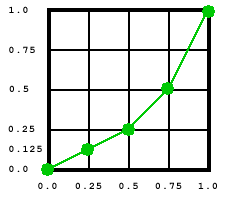The graph above shows a simple 1-input, 1-output function with the domain [0, 1] and range [0, 1], approximated with 5 samples:

f(0.00) = 0.0
f(0.25) = 0.125
f(0.50) = 0.25
f(0.75) = 0.5
f(1.00) = 1.0

For this example, we have chosen the BitsPerSample value of 8. The sample data needs to be recalculated according to the normalized range [0, 2BitsPerSample - 1], or [0, 255], as follows:

(0.0, 0.125, 0.25, 0.5, 1.0) -> (0, 32, 64, 128, 255)

The following code sample implements this function and uses it for grayscale transformation in a Separation color space:

Set Func = doc.CreateFunction("type=0; Dmin1=0;Dmax1=1; Rmin1=0;Rmax1=1; Size1=5; BitsPerSample=8")
Func.SetSampleData Array(0, 32, 64, 128, 255)

Set csGray = doc.CreateColorSpace("DeviceGray")
Set cs = doc.CreateColorSpace("Separation")
cs.SetSeparationParams "PANTONE 360 CV", csGray, Func

If we were to choose the BitsPerSample value of 16 instead, we would need to recalculate the sample values according to the range [0, 65535] and the code would be as follows:

Set Func = doc.CreateFunction("type=0; Dmin1=0;Dmax1=1; Rmin1=0;Rmax1=1; Size1=5; BitsPerSample=16")
Func.SetSampleData Array(0, 8192, 16384, 32768, 65535)
...

Please refer to the previous section of this chapter for more sampled function examples.

Exponential Interpolation Functions

Exponential interpolation (type 2) functions define an exponential interpolation of one input and n output values.

To create an instance of the PdfFunction object representing a type 2 function, the CreateFunction method must be called with the parameter Type=2, and the required domain and optional range parameters for the one input and n output values, as described above. Additionally, a number of required and optional parameters specific to exponential interpolation functions are also used. These are:

• CZero1, CZero2, ..., CZeron (optional) - define the function result when x=0.0. Default values: (0, 0, ..., 0).
• COne1, COne2, ..., COnen (optional) - define the function result when x=1.0. Default values: (1, 1, ..., 1).
• N (required) - the interpolation exponent. Each input value x will return n values computed as follows:
yj = CZeroj + xN * ( COnej - CZeroj)

Example:

Set Param = PDF.CreateParam
Param("Type") = 2
Param("Dmin1") = 0
Param("Dmax1") = 1
Param("Rmin1") = 0
Param("Rmax1") = 1
Param("Rmin2") = 0
Param("Rmax2") = 1
Param("Rmin3") = 0
Param("Rmax3") = 1
Param("CZero1") = 0.2
Param("COne1") = 0.9
Param("CZero2") = 0.2
Param("COne2") = 0.9
Param("CZero3") = 0.2
Param("COne3") = 0.9
Param("N") = 0.6

Set Func = doc.CreateFunction(Param)

Stitching Functions

Stitching (type 3) functions define a stitching of the subdomains of several 1-input functions (referred to as "subfunctions" in this manual) to produce a single new 1-input function. This manual uses the letter k to denote the total number of subfunctions in this stitching function.

To create an instance of the PdfFunction object representing a type 3 function, the CreateFunction method must be called with the parameter Type=3, and the required Domain and optional Range parameters for the one input and n output values, as described above. Additionally, a number of required parameters specific to stitching functions are also used. These are:

• Bounds1, Bounds2, ..., Bounds(k-1) (required) - a group of (k-1) numbers which, in combination with the domain parameters, define the intervals to which each subfunction applies.
• EncodeMin1, EncodeMax1, EncodeMin2, EncodeMax2, ..., EncodeMink, EncodeMaxk (required) - a group of k pairs of numbers which map each subset of the domain defined by the Domain and Bounds parameters to the domain of the corresponding subfunction.

In addition to these parameters, the method AddFunction must be called on this PdfFunction object k times. This method expects a single argument, another instance of the PdfFunction object which represents a subfunction for this stitching function. The output dimensionality of all subfunctions must be the same and match the range parameters of this stitching function, if they are specified.

The Bounds parameters describes a series of half-opened intervals, closed on the left and open on the right (except the last which is closed on the right as well.)

The Encode parameters specify k pairs of numbers. A value x from the ith subdomain is encoded as follows:

x' = Interpolate( x, Boundsi-1, Boundsi, EncodeMini, EncodeMaxi) for i between 1 and k. In this equation, Bounds0 means Dmin1, and Boundsk means Dmax1.

Example:

The following example combines two subfunctions, an exponential-interpolation one and a sampled one, into a single stitching function. Both subfunctions have the domains [0, 1]. The first subfunction is mapped to the subdomain [0, 0.5] of the main function, and the 2nd subfunction to the subdomain [0.5, 1].

Exponential interpolation subfunction: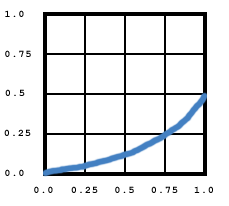Sampled subfunction:Resultant stitching function: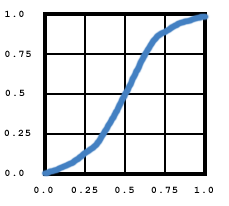Set Func1 = doc.CreateFunction("type=2; Dmin1=0; Dmax1=1; Rmin1=0; Rmax1=1; N=2; CZero1=0; COne1=0.5")

Set Func2 = doc.CreateFunction("type=0; Dmin1=0; Dmax1=1; Rmin1=0; Rmax1=1; Size1=5;BitsPerSample=8")
Func2.SetSampleData Array(125, 191, 223, 231, 255)

Set Func3 = doc.CreateFunction("type=3; Dmin1=0; Dmax1=1; bounds1=0.5; EncodeMin1=0; EncodeMax1=1; EncodeMin2=0; EncodeMax2=1")

PostScript Calculator Functions

A PostScript Calculator (type 4) function is defined as a text string of code written in a small subset of the PostScript language. The detailed description of the commands that can be used in a type 4 function is outside the scope of this manual. Please refer to the Adobe PDF Reference for more information.

To create an instance of the PdfFunction object representing a type 4 function, the CreateFunction method must be called with the parameter Type=4, and the required domain and range parameters for each input and output values, as described above. There are no additional parameters.

An additional step must be taken to make a PdfFunction object representing this type of function valid and complete. A text string containing the actual PostScript code must be specified via the property PostScript.

Example:

Set Func = doc.CreateFunction("Type=4;Dmin1=-1; Dmax1=1; Dmin2=-1; Dmax2=1; Rmin1=-1; Rmax1=1")
Func.PostScript = "{ 360 mul sin 2 div exch 360 mul sin 2 div add }"

## 16.3 Code Sample

This chapter would not be complete without a few PDF-generating VBScript/C# lines of code. The following code sample displays an image in its default form as well as under an ICCBased color space. It also draws a series of bars under the default grayscale color space, a Separation color space with a sampled transformation function, and a calibrated grayscale color space.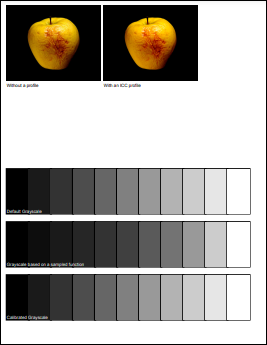Set Pdf = Server.CreateObject("Persits.Pdf")
Set Doc = Pdf.CreateDocument

' Create some color spaces
Set csICC = Doc.CreateColorSpace( "ICCBased", "N=3" )

' Draw an image with the default color space, and the same image with an ICC profile
Set Image = Doc.OpenImage( Server.MapPath( "apple.jpg" ) )
Page.Canvas.DrawImage Image, "x=20; y=600; scalex=0.5, scaley=0.5"
Page.Canvas.DrawText "Without a profile", "x=20; y=595", Doc.Fonts("Helvetica")

Set Image2 = Doc.OpenImage( Server.MapPath( "apple.jpg" ) )
Image2.SetColorSpace csICC
Page.Canvas.DrawImage Image2, "x=240; y=600; scalex=0.5, scaley=0.5"
Page.Canvas.DrawText "With an ICC profile", "x=240; y=595", Doc.Fonts("Helvetica")

' Grayscale color space

Set csGray = doc.CreateColorSpace("DeviceGray")
DrawBars 300, csGray, "Default Grayscale"

' Separation color space with a sampled transformation function
Set Func = doc.CreateFunction("type=0; Dmin1=0;Dmax1=1; Rmin1=0;Rmax1=1; Size1=5; BitsPerSample=8")
Func.SetSampleData Array(0, 32, 64, 128, 255)
Set csSep = doc.CreateColorSpace("Separation")
csSep.SetSeparationParams "PANTONE 4525 C", csGray, Func
DrawBars 180, csSep, "Grayscale based on a sampled function"
' Calibrated Gray
Set csCalGray = doc.CreateColorSpace("CalGray", "Xw=0.7; Yw=1; Zw=1.3")
DrawBars 60, csCalGray, "Calibrated Grayscale"
Filename = Doc.Save( Server.MapPath( "colorspace.pdf" ), False )

Sub DrawBars( YCoord, ColorSpace, Title )
' stroking colors: for bar borders
Page.Canvas.SetColorEx "c1=0"
Page.Canvas.LineWidth = 5

Page.Canvas.SetFillColorSpace ColorSpace

For c = 0 to 1 step 0.1
Page.Canvas.SetFillColorEx "c1=" & c

Page.Canvas.DrawRect 20 + c * 500, YCoord, 50, 100
Page.Canvas.FillRect 20 + c * 500, YCoord, 50, 100
Next

Page.Canvas.DrawText Title, "x=20; y=" & YCoord + 10, Doc.Fonts("Helvetica")
End Sub

IPdfPage objPage;
IPdfDocument objDoc;

{
IPdfManager objPdf = new PdfManager();
objDoc = objPdf.CreateDocument( Missing.Value );
objPage = objDoc.Pages.Add( Missing.Value, Missing.Value, Missing.Value );

// Create some color spaces
IPdfColorSpace csICC = objDoc.CreateColorSpace( "ICCBased", "N=3" );

// Draw an image with the default color space, and the same image with an ICC profile
IPdfImage objImage = objDoc.OpenImage( Server.MapPath( "apple.jpg" ), Missing.Value );
objPage.Canvas.DrawImage( objImage, "x=20; y=600; scalex=0.5, scaley=0.5" );
objPage.Canvas.DrawText( "Without a profile", "x=20; y=595", objDoc.Fonts["Helvetica", Missing.Value] );

IPdfImage objImage2 = objDoc.OpenImage( Server.MapPath( "apple.jpg" ), Missing.Value );
objImage2.SetColorSpace( csICC );
objPage.Canvas.DrawImage( objImage2, "x=240; y=600; scalex=0.5, scaley=0.5" );
objPage.Canvas.DrawText( "With an ICC profile", "x=240; y=595", objDoc.Fonts["Helvetica", Missing.Value] );

// Grayscale color space
IPdfColorSpace csGray = objDoc.CreateColorSpace("DeviceGray", Missing.Value);
DrawBars( 300, csGray, "Default Grayscale" );

// Separation color space with a sampled transformation function
IPdfFunction objFunc = objDoc.CreateFunction("type=0; Dmin1=0;Dmax1=1; Rmin1=0;Rmax1=1; Size1=5; BitsPerSample=8");
object [] arrSamples = new object { (object)0, (object)32, (object)64, (object)128, (object)255 };
objFunc.SetSampleData( arrSamples );
IPdfColorSpace csSep = objDoc.CreateColorSpace("Separation", Missing.Value);
csSep.SetSeparationParams( "PANTONE 4525 C", csGray, objFunc );

DrawBars( 180, csSep, "Grayscale based on a sampled function" );

// Calibrated Gray
IPdfColorSpace csCalGray = objDoc.CreateColorSpace("CalGray", "Xw=0.7; Yw=1; Zw=1.3");
DrawBars( 60, csCalGray, "Calibrated Grayscale" );

String strFilename = objDoc.Save( Server.MapPath("colorspace.pdf"), false );

}

void DrawBars( int YCoord, IPdfColorSpace ColorSpace, string Title )
{
// stroking colors: for bar borders
objPage.Canvas.SetColorEx( "c1=0" );
objPage.Canvas.LineWidth = 5;

objPage.Canvas.SetFillColorSpace( ColorSpace );

for( double c = 0; c <= 1; c += 0.1 )
{
objPage.Canvas.SetFillColorEx( "c1=" + c.ToString() );

objPage.Canvas.DrawRect( 20 + (float)c * 500, YCoord, 50, 100 );
objPage.Canvas.FillRect( 20 + (float)c * 500, YCoord, 50, 100 );
}

objPage.Canvas.DrawText( Title, "x=20; y=" + (YCoord + 10).ToString(), objDoc.Fonts["Helvetica", Missing.Value] );
}

Click the links below to run this code sample:

## 16.4 Using Color Spaces with PdfTable and Other Objects

16.4.1 PdfTable

As of Version 2.7, the PdfTable object has been retrofitted to enable the border and background colors of a table to be specified in color spaces other than RGB (previous versions only allowed the table colors to be in the RGB color space.) To allow color spaces to be specified via PdfParam objects or parameter strings, the color space index, a unique integer identifier, is passed instead of an instance of the PdfColorSpace object.

Two new methods have been added to enable arbitrary colors to be specified: PdfTable.SetColor and PdfCell.SetColor. The former can be used to specify the table border, table background, cell border and cell background colors for the entire table. The latter specifies the border and background colors for an individual cell.

Both SetColor methods expect a single argument: a PdfParam object or parameter string containing the following parameters:

• Type - specifies which color is to be modified. The valid values are: 1 (border color), 2 (cell border color), 3 (background color) and 4 (cell background color). The PdfTable.SetColor method allows all four values, while PdfCell.SetColor only allows values 2 and 4.
• ColorSpace - specifies the color space index. This value should be obtained from the Index property of the PdfColorSpace object.
• c1, c2, ..., cN - specify the individual color components for the color. These values must be in the range [0, 1] and the number of color components must match the color space being used.
• Top, Bottom, Left, Right - if set to True, specify which side(s) of the cell the specified border color should apply to. These parameters are only used by PdfCell.SetColor and only if Type=2. If none of these are set to True, the color applies to the entire cell border.

Starting with Version 2.7, the ColorSpace and c1, c2, ..., cN parameters can also be used with the PdfCanvas.DrawText and PdfCell.AddText methods to specify text colors in color spaces other than RGB and CMYK.

The following code sample creates an ICC-based color space and uses it to paint an entire table, an individual cell, and a text string inside a cell:

Set Pdf = Server.CreateObject("Persits.Pdf")
Set Doc = Pdf.CreateDocument

' Create an ICC color space
Set csICC = Doc.CreateColorSpace( "ICCBased", "N=3" )

Set Table = doc.CreateTable("rows=2; cols=3; width=400; height=300; border=10; cellborder=5; cellspacing=10; cellpadding=2")
Table.Font = Doc.Fonts("Helvetica")

' Border color
Table.SetColor "Type=1; ColorSpace=" & csICC.Index & "; c1=1; c2=0.5; c3=0.2"

' Cell Border Color
Table.SetColor "Type=2; ColorSpace=" & csICC.Index & "; c1=0; c2=0; c3=1"

' BG Color
Table.SetColor "Type=3; ColorSpace=" & csICC.Index & "; c1=1; c2=1; c3=0"

' Cell BG Color
Table.SetColor "Type=4; ColorSpace=" & csICC.Index & "; c1=0; c2=1; c3=0"

' Border color for individual cell
Table(1, 1).SetColor "Type=2; ColorSpace=" & csICC.Index & "; c1=1; c2=0; c3=1"

' Draw text, use the same color space for it
Table(1, 1).AddText "Test", "ColorSpace=" & csICC.Index & ";c1=0.2; c2=0.4; c3=0.3"

' Render table
Page.Canvas.DrawTable Table, "x=106; y=700"

Filename = Doc.Save( Server.MapPath( "cs_table.pdf" ), False )
IPdfManager objPdf = new PdfManager();
objDoc = objPdf.CreateDocument( Missing.Value );

objPage = objDoc.Pages.Add( Missing.Value, Missing.Value, Missing.Value );

// Create an ICC color space
IPdfColorSpace csICC = objDoc.CreateColorSpace( "ICCBased", "N=3" );

IPdfTable objTable = objDoc.CreateTable("rows=2; cols=3; width=400; height=300; border=10; cellborder=5; cellspacing=10; cellpadding=2");
objTable.Font = objDoc.Fonts["Helvetica", Missing.Value];

// Border color
objTable.SetColor("Type=1; ColorSpace=" + csICC.Index + "; c1=1; c2=0.5; c3=0.2");

// Cell Border Color
objTable.SetColor("Type=2; ColorSpace=" + csICC.Index + "; c1=0; c2=0; c3=1");

// BG Color
objTable.SetColor("Type=3; ColorSpace=" + csICC.Index + "; c1=1; c2=1; c3=0");

// Cell BG Color
objTable.SetColor("Type=4; ColorSpace=" + csICC.Index + "; c1=0; c2=1; c3=0");

// Border color for individual cell
objTable[1, 1].SetColor("Type=2; ColorSpace=" + csICC.Index + "; c1=1; c2=0; c3=1");

// Draw text, use the same color space for it
objTable[1, 1].AddText("Test", "ColorSpace=" + csICC.Index + ";c1=0.2; c2=0.4; c3=0.3", Missing.Value);

// Render table
objPage.Canvas.DrawTable( objTable, "x=106; y=700" );

String strFilename = objDoc.Save( Server.MapPath("cs_table.pdf"), false );

Click the links below to run this code sample:

16.4.2 HTML Mode of DrawText Method

As of Version 3.7.0.3, the Canvas.DrawText method, used in the HTML mode (the parameter HTML is set to true), is no longer limited to the DeviceRGB color space. The new parameter ColorComponents, if set to a number from 1 to 3, instructs the DrawText method not to set the current color space to DeviceRGB but to rely on a color space set prior to calling this method. Up to 3 colorants are retrieved from the HTML string's color-setting attributes (such as <font color="#FF0000"> or <font style="color: red">), and converted from the [0 - 255] range to the [0.0 - 1.0] one,

The following code snippet makes the DrawText method draw various pieces of the text in two separate single-colorant colors in the Pantone color space:

Set csRGB = doc.CreateColorSpace("DeviceRGB")
Set objFunc = doc.CreateFunction("type=0; Dmin1=0; Dmax1=1; Rmin1=0; Rmax1=1; Rmin2=0; Rmax2=1; Rmin3=0; Rmax3=1; Size1=2; BitsPerSample=8")
objFunc.SetSampleData (Array(0, 0, 0, 207, 109, 79))
Set csSep = doc.CreateColorSpace("Separation")
csSep.SetSeparationParams "PANTONE 7618 C", csRGB, objFunc

Page.Canvas.SetFillColorSpace csSep

Params = "x=10; y=100; html=true; ColorComponents=1"

Set Font = doc.fonts("Helvetica")
Page.Canvas.DrawText "<font color=""#FF"">Hello</font> <font color=""#A0"">World</font>", Params, Font

Here, the word "Hello" is drawn with the colorant 1 (the Hex value #FF is 255, which converts to 1) and the word "World" with the colorant 0.627451 (the Hex value #A0 is 160, which converts to 0.627451.)

16.5.1 Patterns: Introduction

When a stroking, filling, or text-drawing method is called on the page canvas, such as DrawLine, FillRect, or DrawText, an area of the page is painted with the current color. Usually, a single color is applied which covers the area uniformly. However, it is also possible to apply "paint" that consists of a repeating graphical figure or smoothly varying color gradient instead of a single color. Such a repeating figure or smooth gradient is called a pattern.

Prior to Version 3.5, AspPDF provided limited support for patterns via the method PdfCanvas.FillWithPattern described in Section 5.3.2 - Tiling Patterns. As of Version 3.5, AspPDF's pattern functionality is not limited to tiling patterns: patterns based on shadings, such as smoothly varying color gradients, are also supported. Version 3.5 also allows patterns to be used not only for filling but also stroking operations.

A pattern is created via the method PdfDocument.CreatePattern. Since patterns are conceptually very similar to graphics, a pattern is represented by the PdfGraphics object. Once the pattern is created, it can be passed to the PdfCanvas.SetColorEx and PdfCanvas.SetFillColorEx methods to be used in subsequent stroking and filling operations, as if it were a regular color.

The first argument to the CreatePattern method is a list of parameters. For tiling patterns, CreatePattern is called with the parameter Type=1 (tiling pattern). The 2nd argument is set to Nothing (null). For shading patterns, the method is called with the parameter Type=2 (shading pattern), and the 2nd argument set to an instance of the PdfShading object creatable via the PdfDocument.CreateShading method.

The following drawing is generated by the script shown below. The top portion of the symbol is filled with a tiling pattern based on an image. The lower portion is filled with a shading pattern based on an axial red-to-blue gradient. The parameters used in the script below are explained in detail in the following sub-section.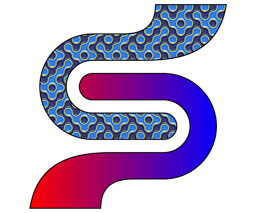Set PDF = Server.CreateObject("Persits.PDF")
Set doc = PDF.CreateDocument

' Create tiling pattern (type=1) based on an image. Set it as fill color
Set Image = doc.OpenImage(Server.MapPath("pattern.jpg"))
Set Pattern = doc.CreatePattern("type=1; left=0; bottom=0; right=64; top=64", Nothing)
Pattern.Canvas.DrawImage Image, "x=0; y=0; scalex=0.1; scaley=0.1"
Page.Canvas.SetFillColorEx Pattern

With Page.Canvas
.SetCTM 2, 0, 0, 2, 350, 350
.MoveTo 0, 0
.AddCurve 7.243, 0, 13.115, -5.872, 13.115, -13.115
.AddCurve 13.115, -20.358, 7.243, -26.23, 0, -26.23
.LineTo -70.764, -26.23
.AddCurve -85.201, -26.23, -98.321, -20.331, -107.822, -10.83
.AddCurve -117.324, -1.328, -123.223, 11.791, -123.223, 26.229
.AddCurve -123.223, 55.038, -99.572, 78.688, -70.764, 78.688
.LineTo -41.599, 78.688
.AddCurve -23.442, 78.688, -8.141, 83.7, -8.141, 107.869
.LineTo 62.296, 107.87
.AddCurve 53.559, 53.31, 5.894, 52.458, -24.853, 52.458
.LineTo -70.764, 52.458
.AddCurve -85.235, 52.458, -96.992, 40.701, -96.992, 26.229
.AddCurve -96.992, 19.03, -94.037, 12.473, -89.278, 7.714
.AddCurve -84.52, 2.956, -77.963, 0, -70.764, 0
.ClosePath
.FillStroke
End With

Set ColorSpace = doc.CreateColorSpace("DeviceRGB") ' RGB color space
Set Shading = doc.CreateShading("type=2; x0=85; y0=0; x1=460; y1=0; offset1=0; color1=red; offset2=1; color2=blue", Nothing, ColorSpace)
Set Pattern2 = doc.CreatePattern("type=2; left=0; bottom=0; right=300; top=960", Shading)
Page.Canvas.SetFillColorEx Pattern2

With Page.Canvas
.SetCTM 1, 0, 0, 1, -70.76, 13.11
.MoveTo 0, 0
.AddCurve -7.243, 0, -13.115, 5.872, -13.115, 13.115
.AddCurve -13.115, 20.358, -7.243, 26.23, 0, 26.23
.LineTo 70.763, 26.23
.AddCurve 85.202, 26.23, 98.322, 20.332, 107.822, 10.831
.AddCurve 117.324, 1.329, 123.222, -11.792, 123.222, -26.229
.AddCurve 123.222, -55.039, 99.573, -78.688, 70.763, -78.688
.LineTo 41.6, -78.688
.AddCurve 23.442, -78.688, 8.142, -83.7, 8.142, -107.869
.LineTo -62.296, -107.869
.AddCurve -53.559, -53.309, -5.894, -52.458, 24.854, -52.458
.LineTo 70.763, -52.458
.AddCurve 85.235, -52.458, 96.992, -40.702, 96.992, -26.229
.AddCurve 96.992, -19.029, 94.037, -12.472, 89.279, -7.714
.AddCurve 84.521, -2.955, 77.963, 0, 70.763, 0

.ClosePath
.FillStroke
End With

Filename = Doc.Save( Server.MapPath( "pattern.pdf" ), False )
IPdfManager objPdf = new PdfManager();
IPdfDocument objDoc = objPdf.CreateDocument( Missing.Value );
IPdfPage objPage = objDoc.Pages.Add( Missing.Value, Missing.Value, Missing.Value );

// Create tiling pattern (type=1) based on an image. Set it as fill color
IPdfImage objImage = objDoc.OpenImage(Server.MapPath("pattern.jpg"));
IPdfGraphics objPattern = objDoc.CreatePattern("type=1; left=0; bottom=0; right=64; top=64", null);
objPattern.Canvas.DrawImage( objImage, "x=0; y=0; scalex=0.1; scaley=0.1" );

objPage.Canvas.SetFillColorEx( objPattern ); IPdfCanvas objCanvas = objPage.Canvas;
objCanvas.SetCTM( 2, 0, 0, 2, 350, 350 );
objCanvas.MoveTo( 0, 0 );
objCanvas.AddCurve( 7.243f, 0f, 13.115f, -5.872f, 13.115f, -13.115f );
objCanvas.AddCurve( 13.115f, -20.358f, 7.243f, -26.23f, 0f, -26.23f );
objCanvas.LineTo( -70.764f, -26.23f );
objCanvas.AddCurve( -85.201f, -26.23f, -98.321f, -20.331f, -107.822f, -10.83f );
objCanvas.AddCurve( -117.324f, -1.328f, -123.223f, 11.791f, -123.223f, 26.229f );
objCanvas.AddCurve( -123.223f, 55.038f, -99.572f, 78.688f, -70.764f, 78.688f );
objCanvas.LineTo( -41.599f, 78.688f );
objCanvas.AddCurve( -23.442f, 78.688f, -8.141f, 83.7f, -8.141f, 107.869f );
objCanvas.LineTo( 62.296f, 107.87f );
objCanvas.AddCurve( 53.559f, 53.31f, 5.894f, 52.458f, -24.853f, 52.458f );
objCanvas.LineTo( -70.764f, 52.458f );
objCanvas.AddCurve( -85.235f, 52.458f, -96.992f, 40.701f, -96.992f, 26.229f );
objCanvas.AddCurve( -96.992f, 19.03f, -94.037f, 12.473f, -89.278f, 7.714f );
objCanvas.AddCurve( -84.52f, 2.956f, -77.963f, 0f, -70.764f, 0 );
objCanvas.ClosePath();
objCanvas.FillStroke();

IPdfColorSpace objColorSpace = objDoc.CreateColorSpace("DeviceRGB"); // RGB color space
IPdfGraphics objPattern2 = objDoc.CreatePattern("type=2; left=0; bottom=0; right=300; top=960", objShading);
objPage.Canvas.SetFillColorEx( objPattern2 );

objCanvas.SetCTM( 1, 0, 0, 1, -70.76f, 13.11f );
objCanvas.MoveTo( 0, 0 );
objCanvas.AddCurve( -7.243f, 0f, -13.115f, 5.872f, -13.115f, 13.115f );
objCanvas.AddCurve( -13.115f, 20.358f, -7.243f, 26.23f, 0f, 26.23f );
objCanvas.LineTo( 70.763f, 26.23f );
objCanvas.AddCurve( 85.202f, 26.23f, 98.322f, 20.332f, 107.822f, 10.831f );
objCanvas.AddCurve( 117.324f, 1.329f, 123.222f, -11.792f, 123.222f, -26.229f );
objCanvas.AddCurve( 123.222f, -55.039f, 99.573f, -78.688f, 70.763f, -78.688f );
objCanvas.LineTo( 41.6f, -78.688f );
objCanvas.AddCurve( 23.442f, -78.688f, 8.142f, -83.7f, 8.142f, -107.869f );
objCanvas.LineTo( -62.296f, -107.869f );
objCanvas.AddCurve( -53.559f, -53.309f, -5.894f, -52.458f, 24.854f, -52.458f );
objCanvas.LineTo( 70.763f, -52.458f );
objCanvas.AddCurve( 85.235f, -52.458f, 96.992f, -40.702f, 96.992f, -26.229f );
objCanvas.AddCurve( 96.992f, -19.029f, 94.037f, -12.472f, 89.279f, -7.714f );
objCanvas.AddCurve( 84.521f, -2.955f, 77.963f, 0f, 70.763f, 0 );

objCanvas.ClosePath();
objCanvas.FillStroke();

string strFilename = objDoc.Save( Server.MapPath("pattern.pdf"), false );

Click the links below to run this code sample:

16.5.2 Tiling Patterns

A tiling pattern consists of a small graphical figure called a pattern cell. Painting with the pattern replicates the cell at fixed horizontal and vertical intervals to fill an area. The pattern cell can include graphical elements such as filled areas, text, and images.

A pattern cell is a self-contained graphical entity conceptually very similar to a graphics, and is therefore represented by the PdfGraphics object. An instance of the PdfGraphics object representing a pattern cell is created via the PdfDocument.CreatePattern method, which accepts two arguments: a list of parameters, and an instance of the PdfShading object (described below). For a tiling pattern, the 2nd argument must be set to Nothing (null). Once an instance of the pattern object is created, arbitrary painting methods are called on its canvas to form the graphical figure for the pattern, such as PdfCanvas.DrawLine, PdfCanvas.FillRect, PdfCanvas.DrawText, etc. The pattern object can then be passed to the PdfCanvas.SetColorEx and PdfCanvas.SetFillColorEx methods as if it were a regular color.

The pattern-creating parameters passed via the 1st argument to the CreatePattern method are as follows:

• Type (required) -- must be set to 1 for a tiling pattern.
• Left, Bottom, Right, Top (required) -- specify the coordinates, in the pattern's coordinate system, of the left, bottom, right and top edges, respectively.
• PaintType (optional) -- 1 for a colored tiling pattern, 2 for an uncolored tiling pattern. 1 (colored pattern) is the default value. An uncolored pattern does not specify any color information. Instead, the entire pattern cell is painted with a separately specified color each time the pattern is used. Essentially, it describes a stencil through which the current color is to be poured.
• TilingType (optional) -- a code that controls adjustments to the spacing of the tiles relative to the device pixel grid. The valid values are: 1 (default) - constant spacing; 2 - no distortion; 3 - constant spacing and faster tiling.
• XStep, YStep (optional) -- the desired horizontal and vertical spacing between pattern cells, respectively, measured in the pattern coordinate system. Right - Left and Top - Bottom by default, respectively.
• a, b, c, d, e, f (optional) -- specify the pattern matrix. The identity matrix [1, 0, 0, 1, 0, 0] by default.

A simple tiling pattern based on a single image, such as the one used in the code sample above, can be created as follows:

' Open a 640x640 image
Set Image = doc.OpenImage(Server.MapPath("pattern.jpg"))

' Create a 64x64 tiling pattern cell
Set Pattern = doc.CreatePattern("type=1; left=0; bottom=0; right=64; top=64", Nothing)

' Scale the image down to fit the 64x64 pattern cell
Pattern.Canvas.DrawImage Image, "x=0; y=0; scalex=0.1; scaley=0.1"

' Use the pattern as a fill color
Page.Canvas.SetFillColorEx Pattern

Shading patterns provide a smooth transition between colors across an area to be painted, independent of the resolution of any particular output device and without specifying the number of steps in the color transition.

A shading pattern is created via the PdfDocument.CreatePattern method with the 2nd argument set to an instance of the PdfShading object described in detail in the next sub-section. The pattern-creating parameters passed via the 1st argument to the CreatePattern method are as follows:

• Type (required) -- must be set to 2 for a shading pattern.
• Left, Bottom, Right, Top (required) -- specify the coordinates, in the pattern's coordinate system, of the left, bottom, right and top edges, respectively.
• a, b, c, d, e, f (optional) -- specify the pattern matrix. The identity matrix [1, 0, 0, 1, 0, 0] by default.

Once a shading pattern object is created, it can optionally be associated with a graphics state object (PdfGState) via the method PdfGraphics.SetGState. This PdfGState object is put into effect temporarily while the shading pattern is painted. Graphics state objects are covered in Section 19.1 - PdfGState Object: Overview.

Just like a tiling pattern object, a shading pattern object can be passed to the PdfCanvas.SetColorEx and PdfCanvas.SetFillColorEx methods as if it were a regular color.

The following code sample creates a simple radial shading, then a shading pattern based on that shading, and paints the letter "A" with it. The detailed explanation of the parameters used to create the shading is provided below.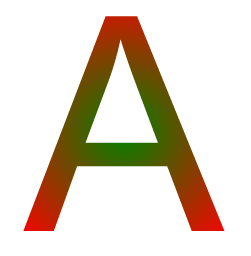' Create RGB color space
Set ColorSpace = doc.CreateColorSpace("DeviceRGB")

Set Shading = doc.CreateShading("type=3; x0=200; y0=200; r0=0; x1=200; y1=200; r1=150; offset1=0; color1=green; offset2=1; color2=red", Nothing, ColorSpace)

Set pattern = doc.CreatePattern("type=2; left=0; bottom=0; right=300; top=960", Shading)

' Draw the letter A using the pattern as the fill color
Page.Canvas.SetFillColorEx pattern
Page.Canvas.DrawText "A", "x=100; y=400; size=300", doc.fonts("Helvetica-Bold")

A shading is represented by the PdfShading object creatable by the PdfDocument.CreateShading method which expects three arguments: the list of parameters, a PdfFunction object, and a PdfColorSpace object. An instance of the PdfShading object can then be used to create a shading pattern by passing it to the CreatePattern method as the 2nd argument, or directly paint with it via the PdfCanvas.FillWithShading method.

The PdfColorSpace object can represent any color space described earlier in this chapter. The PdfFunction object must represent a function with the number of outputs corresponding to the number of colorants in the specified color space. For example, if the DeviceRGB color space is specified, the number of outputs in the specified function must be 3. The number of inputs depends on the shading type and must be either 1 or 2. The PdfColorSpace argument is required for all shading types. The PdfFunction argument is required for some shading types but not others.

AspPDF currently supports three types of shading: Function-based, Axial and Radial. Each type is described in detail below.

In function-based (type 1) shadings, the color at every point in the domain is defined by a specified mathematical function. Mathematical functions and the PdfFunction object representing them are described in detail earlier in this chapter in Section 16.2 - PdfFunction Object.

The function must have 2 inputs, and the number of outputs matching the number of colorants of the specified color space.

The shading-creating parameters passed via the 1st argument to the CreateShading method are as follows:

• Type (required) -- must be 1 for function-based shadings.
• bc1, bc2, ..., bcN (optional) -- the color components for the background color. N is the number of colorants in the specified color space. If specified, this color is used to fill the area before any painting operations involving the shading are applied.
• XMin, YMin, XMax, YMax (optional) -- specify the rectangular domain of coordinates over which the color function is defined. [0.0, 1.0, 0.0, 1.0] by default.
• Left, Bottom, Right, Top (optional) -- specify the shading's bounding box. The coordinates are interpreted in the shading's target coordinate space. If present, this box is applied as a temporary clipping boundary when the shading is painted.
• a, b, c, d, e, f (optional) -- specify the transformation matrix mapping the coordinate space specified by the domain [XMin, YMin, XMax, YMax] into the shading's target coordinate space. The identity matrix [1, 0, 0, 1, 0, 0] by default.
• AntiAlias (optional) -- a Boolean flag indicating whether to filter the shading function to prevent antialiasing artifacts. False by default.

For example, let's create a simple function-based shading over a rectangle with the corner colors (in RGB color space) are as follows:

• lower-left: yellow (rgb 1, 1, 0);
• lower-right: black (rgb 0, 0, 0);
• upper-left: red (rgb 1, 0, 0);
• upper-right: blue (rgb 0, 0, 1).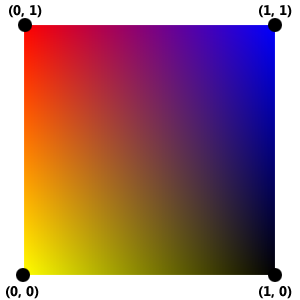To implement this shading, we need to create a function with two inputs (x and y coordinates) and three outputs (R, G, and B colorants). The sampled function (Type=0) is suitable for this task. The sample values are:

• f(0, 0) = [1, 1, 0] (yellow, lower-left corner)
• f(1, 0) = [0, 0, 0] (black, lower-right corner)
• f(0, 1) = [1, 0, 0] (red, upper-left corner)
• f(1, 1) = [0, 0, 1] (blue, upper-right corner)

We will set BitsPerSample parameter in our function to 8. All 0s in our sampled values remain 0s, and all 1s become 255. The sample values need to be listed according to the rule "the sample values in the first dimension vary fastest, and the values in the last dimension vary slowest" (see sub-section 16.2.3 above), as follows: f(0, 0), f(1, 0), f(0, 1), f(1, 1), or [ [255, 255, 0], [0, 0, 0], [255, 0, 0], [0, 0, 255] ].

The following script creates the shading above:

Set ColorSpace = doc.CreateColorSpace("DeviceRGB")
Set Func = doc.CreateFunction("type=0; Dmin1=0; Dmax1=1; Dmin2=0; Dmax2=1; rmin1=0; rmax1=1; rmin2=0; rmax2=1; rmin3=0; rmax3=1; bitspersample=8; size1=2; size2=2")
Func.SetSampleData Array(255, 255, 0, 0, 0, 0, 255, 0, 0, 0, 0, 255)

However, if we were to attempt to use this shading directly to paint an area of the page with the FillWithShading method, we would discover that the area remains unpainted. That is because the shading has been created over a tiny square with the dimensions 1x1 (in user units). To fill a large area, we need to stretch the shading and also shift it via the a, b, c, d, e, f parameters. The following snippet does just that and the output is shown below:

...
Set Shading = doc.CreateShading("type=1; a=200; b=0; c=0; d=400; e=50; f=150", Func, ColorSpace)

Page.Canvas.ClosePathThe following snippet associates the shading with a pattern and uses this pattern to paint the letter "A". The result is shown below:

...
Set Shading = doc.CreateShading("type=1; a=200; b=0; c=0; d=400; e=50; f=150", Func, ColorSpace)

Set Pattern = doc.CreatePattern("type=2; left=0; bottom=0; right=300; top=960", Shading)
Page.Canvas.SetFillColorEx Pattern
Page.Canvas.DrawText "A", "x=50; y=500; size=250", doc.fonts("Helvetica-Bold")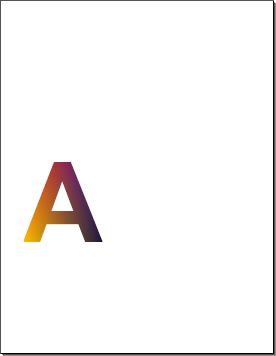Axial shadings define a color blend that varies along a linear axis between two endpoints and extends indefinitely perpendicular to this axis. The shading may optionally be extended beyond either or both endpoints by continuing the boundary colors indefinitely.

The shading-creating parameters for axial shadings passed via the 1st argument to the CreateShading method are as follows:

• Type (required) -- must be 2 for axial shadings.
• X0. Y0, X1, Y1 (required) -- specify the starting and ending coordinates of the axis, expressed in the shading's target coordinate space.
• Offset1, Color1, Offset2, Color2, ..., OffsetM, ColorM (optional) -- a set of pairs Offset/Color defining a gradient. Offsets must be in the range [0.0, 1.0]. If at least two pairs are specified, the Function argument to the CreateShading method is not used and ignored. See below for more information.
• ExtendStart, ExtendEnd (optional) -- two Boolean flags specifying whether to extend the shading beyond the starting and ending points of the axis, respectively. (False, False) by default.
• bc1, bc2, ..., bcN (optional) -- the color components for the background color. N is the number of colorants in the specified color space. If specified, this color is used to fill the area before any painting operations involving the shading are applied.
• t0, t1 (optional) -- specify the limiting values of a parametric variable t which is the input argument to the color function. [0.0, 1.0] by default.
• Left, Bottom, Right, Top (optional) -- specify the shading's bounding box. The coordinates are interpreted in the shading's target coordinate space. If present, this box is applied as a temporary clipping boundary when the shading is painted.
• AntiAlias (optional) -- a Boolean flag indicating whether to filter the shading function to prevent antialiasing artifacts. False by default.

The Function argument to the CreateShading method must be set to an instance of the PdfFunction object representing a function with one input and the number of outputs matching the number of colorants in the specified color space. Instead of passing a PdfFunction object, a set of pairs Offset/Color can be specified defining a color gradient, as shown below.

To demonstrate a simple axial shading, let's create a shading with the appearance of a horizontal red-to-blue gradient. We will use a sample function again with the following sample values:

• f(0) = [1, 0, 0] (red)
• f(1) = [0, 0, 1] (blue)

The gradient will extend horizontally from the points (100, 0) to (500, 0).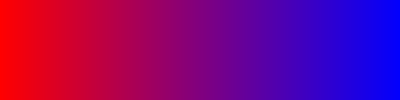' Sample function with one input and 3 outputs
Set Func = doc.CreateFunction("type=0; Dmin1=0; Dmax1=1; rmin1=0; rmax1=1; rmin2=0; rmax2=1; rmin3=0; rmax3=1; bitspersample=8; size1=2;")
Func.SetSampleData Array(255, 0, 0, 0, 0, 255) ' Red to Blue

' RGB color space
Set ColorSpace = doc.CreateColorSpace("DeviceRGB")

' Create axial shading and fill a rectangle with it

Page.Canvas.ClosePath
Page.Canvas.Clip ' to limit the shading area to this rectangle only

The simple two-color gradient shown above can be easily defined by a single sample function. However, if the gradient consists of multiple colors and arbitrary distances between the key color positions, a stitching function comprised of multiple sample functions would be needed, and the coding would be more complex. The CreateShading method is capable of creating multi-color gradients when it is passed a list of pairs Offset/Color specifying the offset and color of all key positions of the gradient. All offsets must be in the range [0, 1], and must be specified in an ascending order.

The simple red-to-blue gradient above can be created with two Offset/Color pairs and without the use of a function, as follows:

...
Set Shading = doc.CreateShading("type=2; x0=100; y0=0; x1=500; y1=0; Offset1=0; Color1=red; Offset2=1 Color2=blue;", Nothing, ColorSpace)

A more complex multi-color gradient with unevenly spaced color positions such as the this one, can be created as follows: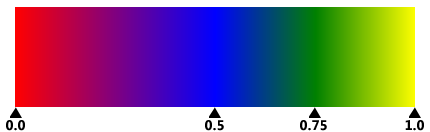...
Set Shading = doc.CreateShading("type=2; x0=100; y0=0; x1=500; y1=0; Color1=red; Offset1=0; Color2=blue; Offset2=0.5; Color3=green; offset3=0.75; Color4=yellow; Offset4 = 1", Nothing, ColorSpace)

Radial shadings define a color blend that varies between two circles. Shadings of this type are commonly used to depict three-dimensional spheres and cones.

• X0. Y0, R0, X1, Y1, R1 (required) -- specify the centers and radii of the starting and ending circles in the shading's target coordinate space.
• The other parameters are exactly the same as in axial shadings, including the Offset/Color pairs.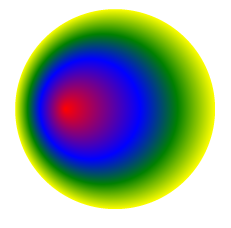The radial shading shown above is based on the same Offset/Color series as the axial shading shown in the previous example. It was created with the following code:

Set ColorSpace = doc.CreateColorSpace("DeviceRGB")
Set Shading = doc.CreateShading("type=3; x0=150; y0=200; r0=0; x1=200; y1=200; r1=100; Color1=red; Offset1=0; Color2=blue; Offset2=0.5; Color3=green; offset3=0.75; Color4=yellow; Offset4 = 1", Nothing, ColorSpace)